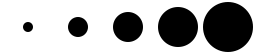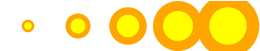# Drawing SVGs

Now that we’re familiar with the basic structure of an SVG image and its elements, how can we start generating shapes from our data?

You may have noticed that all properties of SVG elements are specified as attributes. That is, they are included as property/value pairs within each element tag, like this:

```<element property="value"/>

```

Hmm, that looks strangely like HTML!

```<p class="eureka">

```

We have already used D3’s handy `append()` and `attr()` methods to create new HTML elements and set their attributes. Since SVG elements exist in the DOM, just as HTML elements do, we can use `append()` and `attr()` in exactly the same way to generate SVG images!

## Create the SVG

First, we need to create the SVG element in which to place all our shapes.

```d3.select("body").append("svg");

```

That will find the `body` and append a new `svg` element just before the closing `</body>` tag. While that will work, I recommend:

```var svg = d3.select("body").append("svg");

```

Remember how most D3 methods return a reference to the DOM element on which they act? By creating a new variable `svg`, we are able to capture the reference handed back by `append()`. Think of `svg` not as a “variable” but as a “reference pointing to the SVG object that we just created.” This reference will save us a lot of code later. Instead of having to search for that SVG each time — as in `d3.select("svg")` — we just say `svg`.

```svg.attr("width", 500)

.attr("height", 50);

```

Alternatively, that could all be written as one line of code:

```var svg = d3.select("body")

.append("svg")
.attr("width", 500)
.attr("height", 50);

```

See the demo for that code. Inspect the DOM and notice that there is, indeed, an empty SVG element.

To simplify your life, I recommend putting the width and height values into variables at the top of your code, like this (view the source):

```//Width and height

var w = 500;
var h = 50;

```

I’ll be doing that with all future examples. By variabalizing the size values, they can be easily referenced throughout your code, as in:

```var svg = d3.select("body")

.append("svg")
.attr("width", w) // <-- Here
.attr("height", h); // <-- and here!

```

Also, if you send me a petition to make “variabalize” a real word, I will gladly sign it.

## Data-driven Shapes

Time to add some shapes. I’ll bring back our trusty old data set

```

var dataset = [ 5, 10, 15, 20, 25 ];

```

and then use `data()` to iterate through each data point, creating a `circle` for each one:

```

svg.selectAll("circle")

.data(dataset)
.join("circle");

```

Remember, `selectAll()` will return empty references to all `circle`s (which don’t exist yet), `data()` binds our data to the elements we’re about to create, and `join()` finally adds a `circle` to the DOM.

To make it easy to reference all of the `circle`s later, we can create a new variable to store references to them all:

```

var circles = svg.selectAll("circle")

.data(dataset)
.join("circle");

```

Great, but all these circles still need positions and sizes. Be warned: The following code may blow your mind.

```

circles.attr("cx", function(d, i) {

return (i * 50) + 25;
})
.attr("cy", h/2)
.attr("r", function(d) {
return d;
});

```Feast your eyes on the demo. Let’s step through the code.

```

circles.attr("cx", function(d, i) {

return (i * 50) + 25;
})

```

Takes the reference to all `circle`s and sets the `cx` attribute for each one. Our data has already been bound to the `circle` elements, so for each `circle`, the value `d` matches the corresponding value in our original data set (5, 10, 15, 20, or 25). Another value, `i`, is also automatically populated for us. `i` is a numeric index value of the current element. Counting starts at zero, so for our “first” circle `i == 0`, the second circle’s `i == 1` and so on. We’re using `i` to push each subsequent circle over to the right, because each subsequent loop through, the value of `i` increases.

```

(0 * 50) + 25 returns 25

(1 * 50) + 25 returns 75
(2 * 50) + 25 returns 125
(3 * 50) + 25 returns 175
(4 * 50) + 25 returns 225

```

To make sure `i` is available to your custom function, you must include it as an argument in the function definition (`function(d, i)`). You must also include `d`, even if you don’t use `d` within your function (as in the case above).

On to the next line.

```

.attr("cy", h/2)

```

`h` is the height of the entire SVG, so `h/2` is one-half of its height. This has the effect of aligning all `circle`s in the vertical center.

```

.attr("r", function(d) {

return d;
});

```

Finally, the radius `r` of each `circle` is simply set to `d`, the corresponding data value.

## Pretty Colors, Oooh!

Color fills and strokes are just other attributes which you can set using the same methods. Simply by appending this code

```

.attr("fill", "yellow")

.attr("stroke", "orange")
.attr("stroke-width", function(d) {
return d/2;
});

```

we get the following (see demo):Of course, you can mix and match attributes and custom functions to apply any combination of properties. The trick with data visualization, of course, is choosing appropriate mappings, so the visual expression of your data is understandable and useful for the viewer.

Next up: Types of data